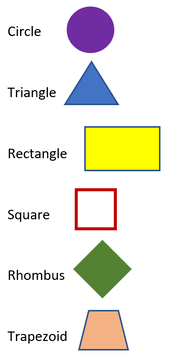# Math Worksheets Land

Math Worksheets For All Ages

# Math Worksheets Land

Math Worksheets For All Ages

# Attributes of Shapes Worksheets

What Are the Attributes of Shapes? To understand what shapes are, we do not need to search for them on the internet as everything around us has a geometric form. Look around; you will see many simple shapes such as square, circle, rectangle, sphere, cylinder, etc. To develop an understanding of all the different forms, it is better to learn their attributes. The properties and traits of any shape are known as its attributes. Let's understand what these attributes are: Features or properties that visually define any form are its attributes. For example: Square is a basic geometric shape. Its attributes will be: Attributes:- It has four sides and four vertices. - All sides of the square are equal. - Opposite sides are parallel. - The angles between any two adjacent sides are 90 degrees. Let's take an equilateral triangle Attributes: - All three sides are equal. - The angle between two adjacent sides is 60 degrees. These worksheets help students identify and get comfortable with geometric forms of all kind.

### Aligned Standard: Grade 1 Geometry - G.1

• Naming Process Step-by-step Lesson- You are given 5 shapes and we show you how to go about naming them properly based on their properties.
• Fill In The Form Guided Lesson - We give you 5 shapes and you just need to figure out which meet the criteria that is given. Some problems will have you find sums and differences, others will have you color specific forms.
• Colored In Guided Lesson Explanation - We walk you through how to identify the proper geometric pattern that you are asked to find.
• What Are There? - Look in the cookie jar and see what shapes are in it. Write down how many of each you see in the cookie jar and create a color code for them.
• Sorting Lesson - A nice break down on shapes for you. You will need four colors here.
• Lots To Identify - Shade in or color the shape we are looking for. It also helps to write how many of each there are, when you are done.
• Working with Different Orientations - Match the shapes that have been rotated or skewed in one way or another a bit.
• Answer Keys - These are for all the unlocked materials above.

### Guided Lessons

My true goal here is for students to start seeing the shapes that surround them all day long.

### Practice Worksheets

We focus on the names of the shapes in all types of settings.

### The Fundamental Geometric Shapes and Their Key FeaturesGeometric shapes are closed two-dimensional figures. What separates one from the next are curves, points, and lines. These things create, in some cases, angles, corners, and sides. There are six commonly held shapes that we often study and set the foundation for most our knowledge of geometry. They also are in many forms of architecture and manufacturing of just about everything. Take a look at what separates each of these figures from another.

Circle - This is a round planar figure. The round curves are always an equal distance from the center.

Triangle - This is formed when three straight lines come together and form three angles. The length of these sides can vary greatly. Mathematicians realized that these sides and angles are always in a consistent proportion to one another. This simple concept created many different complex applications that it created a new branch of mathematics called trigonometry.

Rectangle - This figure has four sides with the opposite sides being equal in length. They also have four angles that measure ninety degrees.

Square -This is a rectangle that has four equal sides and angles.

Rhombus - Formed by four straight lines that measure all the same length. They do not contain any ninety-degree angles. Opposite angles are equal.

Trapezoid - This is a four-sided figure that has two sides that are parallel to one another. The other side pair are not parallel.

Unlock all the answers, worksheets, homework, tests and more!
Save Tons of Time! Make My Life Easier Now

## Thanks and Don't Forget To Tell Your Friends!

I would appreciate everyone letting me know if you find any errors. I'm getting a little older these days and my eyes are going. Please contact me, to let me know. I'll fix it ASAP.